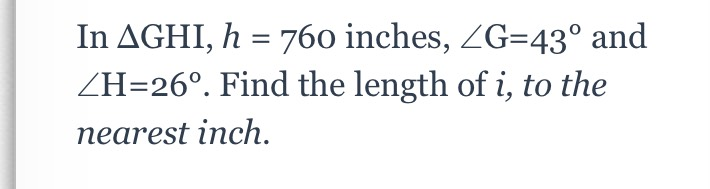### Still have math questions?

Trigonometry
QuestionIn $$\Delta GHI , h = 760$$ inches, $$\angle G = 43 ^ { \circ }$$ and

$$\angle H = 26 ^ { \circ } .$$ Find the length of $$i ,$$ to the nearest inch.